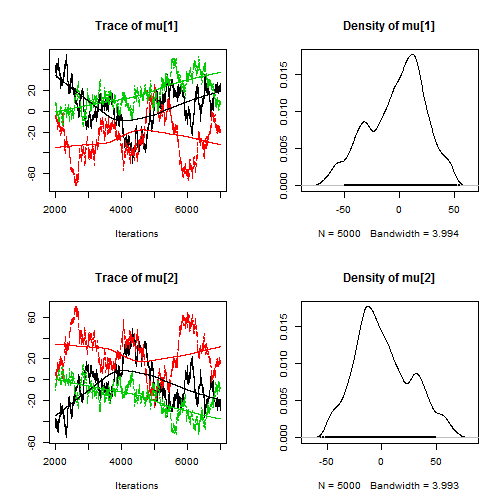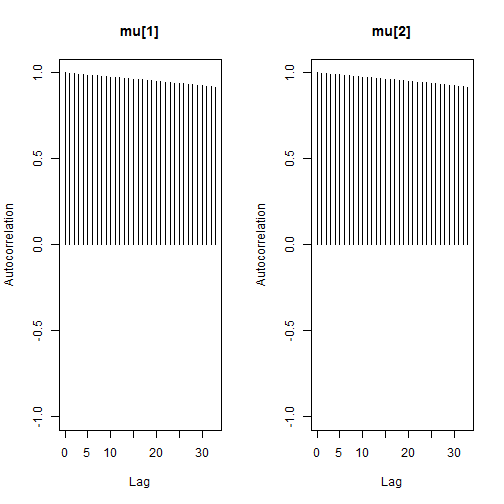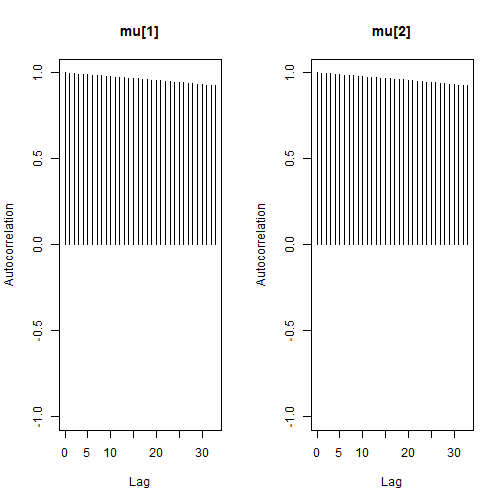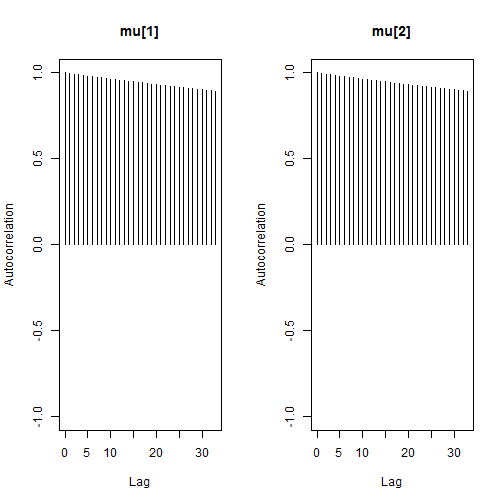# Convergence diagnostics for a ill-posed model

### Chapter 3.4: Diagnosing and improving convergence

In this example the chains do not converge and we show how the convergence diagnostics flag non-convergence. The model is contrived to give poor convergence. It is $Y\sim\mbox{Poisson}(\exp[\mu_1+\mu_2]) \mbox{ where }\mu_1,\mu_2\sim\mbox{Normal}(0,1000).$ This is a silly model because there is only one observation, $$Y=1$$, and two parameters. Further, the two parameter give the same likelihood for all combinations of $$\mu_1$$ and $$\mu_2$$ that give the same sum.

## Define the model as a string

 model_string <- textConnection("model{
Y     ~ dpois(exp(mu+mu))
mu ~ dnorm(0,0.001)
mu ~ dnorm(0,0.001)
}")


## Generate posterior samples

 inits <- list(mu=rnorm(2,0,5))
data  <- list(Y=1)
model <- jags.model(model_string,data = data, inits=inits, n.chains=3, quiet=TRUE)

update(model, 1000, progress.bar="none")
samples <- coda.samples(model,
variable.names=c("mu"),
n.iter=5000, progress.bar="none")


## Graphical diagnostics

The trace plots are meandering, the chains (each chain is a different color) give different estimates, and the autocorrelation is high even for lag 35. All of these indicate poor convergence.

  plot(samples)autocorr.plot(samples)## Numerical diagnostics

  # Autocorrelation near 1 indicates poor convergence
autocorr(samples[],lag=1)

## , , mu
##
##           mu      mu
## Lag 1 0.9969476 -0.9957215
##
## , , mu
##
##            mu     mu
## Lag 1 -0.9975801 0.9970195

  # Low ESS indicates poor convergence
effectiveSize(samples)

##    mu    mu
## 21.18113 20.71919

  # R greater than 1.1 indicates poor convergence
gelman.diag(samples)

## Potential scale reduction factors:
##
##       Point est. Upper C.I.
## mu       2.01        3.5
## mu       2.01        3.5
##
## Multivariate psrf
##
## 1.8

  # |z| greater than 2 indicates poor convergence
geweke.diag(samples[])

##
## Fraction in 1st window = 0.1
## Fraction in 2nd window = 0.5
##
##  mu  mu
##  3.239 -3.347